The OpenFOAM FoundationpolynomialTransport< Thermo, PolySize > Class Template Reference

Transport package using polynomial functions for mu and kappa. More...

Inheritance diagram for polynomialTransport< Thermo, PolySize >: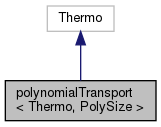[legend]
Collaboration diagram for polynomialTransport< Thermo, PolySize >:[legend]

## Public Member Functions

polynomialTransport (const word &, const polynomialTransport &)
Construct as named copy. More...

polynomialTransport (const dictionary &dict)
Construct from dictionary. More...

autoPtr< polynomialTransportclone () const
Construct and return a clone. More...

scalar mu (const scalar p, const scalar T) const
Dynamic viscosity [kg/m/s]. More...

scalar kappa (const scalar p, const scalar T) const
Thermal conductivity [W/m/K]. More...

scalar alphah (const scalar p, const scalar T) const
Thermal diffusivity of enthalpy [kg/m/s]. More...

void write (Ostream &os) const
Write to Ostream. More...

void operator+= (const polynomialTransport &)

void operator*= (const scalar)

## Static Public Member Functions

static autoPtr< polynomialTransportNew (const dictionary &dict)

static word typeName ()
Return the instantiated type name. More...

## Friends

polynomialTransport operator+ (const polynomialTransport &, const polynomialTransport &)

polynomialTransport operator* (const scalar, const polynomialTransport &)

Ostreamoperator (Ostream &, const polynomialTransport &)

## Detailed Description

### template<class Thermo, int PolySize = 8> class Foam::polynomialTransport< Thermo, PolySize >

Transport package using polynomial functions for mu and kappa.

Usage
Property Description
muCoeffs<8> Dynamic viscosity polynomial coefficients
kappaCoeffs<8> Thermal conductivity polynomial coefficients

Example of the specification of the transport properties:

    transport
{
muCoeffs<8>     ( 1000 -0.05 0.003 0 0 0 0 0 );
kappaCoeffs<8>  ( 2000 -0.15 0.023 0 0 0 0 0 );
}

The polynomial expressions are evaluated as so: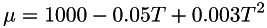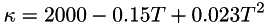Note
• Dynamic viscosity polynomial coefficients evaluate to an expression in [Pa.s], but internally uses [Pa.s/kmol].
• Thermal conductivity polynomial coefficients evaluate to an expression in [W/m/K], but internally uses [W/m/K/kmol].
Source files
Foam::Polynomial

Definition at line 92 of file polynomialTransport.H.

## ◆ polynomialTransport() [1/2]

 polynomialTransport ( const word & name, const polynomialTransport< Thermo, PolySize > & pt )
inline

Construct as named copy.

Definition at line 46 of file polynomialTransportI.H.

## ◆ polynomialTransport() [2/2]

 polynomialTransport ( const dictionary & dict )

Construct from dictionary.

Definition at line 33 of file polynomialTransport.C.

## ◆ clone()

 Foam::autoPtr< Foam::polynomialTransport< Thermo, PolySize > > clone ( ) const
inline

Construct and return a clone.

Definition at line 59 of file polynomialTransportI.H.

## ◆ New()

 Foam::autoPtr< Foam::polynomialTransport< Thermo, PolySize > > New ( const dictionary & dict )
inlinestatic

Definition at line 70 of file polynomialTransportI.H.

References dict, and polynomialTransport< Thermo, PolySize >::mu().

Here is the call graph for this function: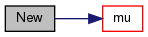## ◆ typeName()

 static word typeName ( )
inlinestatic

Return the instantiated type name.

Definition at line 165 of file polynomialTransport.H.

Here is the call graph for this function: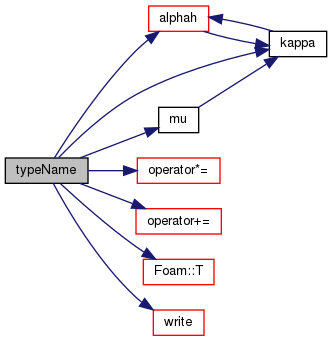## ◆ mu()

 Foam::scalar mu ( const scalar p, const scalar T ) const
inline

Dynamic viscosity [kg/m/s].

Definition at line 83 of file polynomialTransportI.H.

Here is the call graph for this function: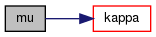Here is the caller graph for this function:## ◆ kappa()

 Foam::scalar kappa ( const scalar p, const scalar T ) const
inline

Thermal conductivity [W/m/K].

Definition at line 94 of file polynomialTransportI.H.

Here is the call graph for this function: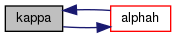Here is the caller graph for this function: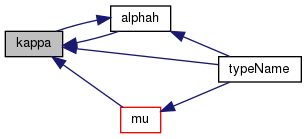## ◆ alphah()

 Foam::scalar alphah ( const scalar p, const scalar T ) const
inline

Thermal diffusivity of enthalpy [kg/m/s].

Definition at line 105 of file polynomialTransportI.H.

References Cp(), and polynomialTransport< Thermo, PolySize >::kappa().

Here is the call graph for this function: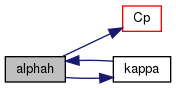Here is the caller graph for this function: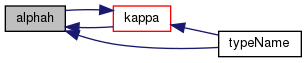## ◆ write()

 void write ( Ostream & os ) const

Write to Ostream.

Definition at line 58 of file polynomialTransport.C.

Referenced by polynomialTransport< Thermo, PolySize >::typeName().

Here is the call graph for this function: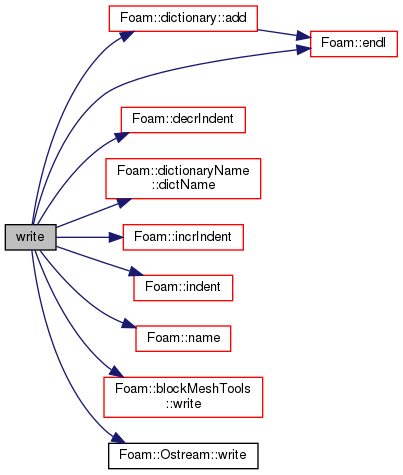Here is the caller graph for this function: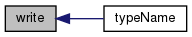## ◆ operator+=()

 void operator+= ( const polynomialTransport< Thermo, PolySize > & )
inline

Definition at line 117 of file polynomialTransportI.H.

References Foam::mag(), and Y.

Referenced by polynomialTransport< Thermo, PolySize >::typeName().

Here is the call graph for this function: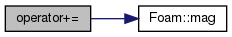Here is the caller graph for this function: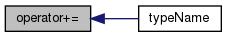## ◆ operator*=()

 void operator*= ( const scalar s )
inline

Definition at line 138 of file polynomialTransportI.H.

References Foam::mag(), and s().

Referenced by polynomialTransport< Thermo, PolySize >::typeName().

Here is the call graph for this function:Here is the caller graph for this function: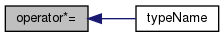## ◆ operator+

 polynomialTransport operator+ ( const polynomialTransport< Thermo, PolySize > & , const polynomialTransport< Thermo, PolySize > & )
friend

## ◆ operator*

 polynomialTransport operator* ( const scalar , const polynomialTransport< Thermo, PolySize > & )
friend

## ◆ operator

 Ostream& operator ( Ostream & , const polynomialTransport< Thermo, PolySize > & )
friend

The documentation for this class was generated from the following files: